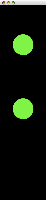## May 14, 2008

### Java Simple PhysicsWell with classes over, I wanted to at least do something with the year of University Physics torture I have endured. Thus I present Java Simple Physics. It is just a quick physics example that uses the kinetic and potential relationship to have balls with different gravity constants drop then bounce with no loss of energy. It took a few hours to get right, mostly because I either did not know what I was doing, or was over complicating things. I hope to slowly improve on this concept till I have a VERY simple physics engine. Hey, it can only help to have a physics engine laying around, one day I might actually need one.

`import javax.swing.*;import java.awt.*;public class Physics extends Thread{ private int worldx = 200, worldy = 900, worldtime = 15; private JFrame frame = new JFrame(); private Ball ball,ball1;    public Physics()     {     // Set up frame     frame.setSize(worldx,worldy);     frame.setVisible(true);     // Make new ball     ball = new Ball(400,450,9.8,worldx,worldy,worldtime);     ball1 = new Ball(400,450,3.8,worldx,worldy,worldtime);    }        public void run() {  while(true)  {   try   {    // Cover old draw with fresh screen    frame.getGraphics().fillRect(0,0,worldx,worldy);        // Move and paint ball    ball.move();    ball.draw(frame.getGraphics());    ball1.move();    ball1.draw(frame.getGraphics());    Thread.sleep(worldtime);       }   catch(Exception e){System.out.print(e);}  } }        public static void main(String args[])    {     Physics p = new Physics();     p.start();    }}class Ball{ private double gravity = 9.8, t; private double x,y,v,a,m,dx,dy,dv,k,u; private int worldx,worldy,dir=-1,width = 90;   public Ball(int xin, int yin, double g, int wx,int wy, int time) {  // Set Constants  worldx = wx; worldy = wy-width; t = time;  // Reduce gravity to fit with drawing refresh rate  gravity = g * .02;    // Center ba;; on x axis  x = worldx/2 + width/2;    // Set varables  y = yin;  v = 0;  a = 0;  m=.8;    // Preset u and k values based on varables  k = (m * gravity * (worldy - width)) - (m * gravity * y);  if(k < 0){ k = 0; }  u = m * gravity * y; }  // Catch and reverse public void move() {  // Calculate u and k  k += u - (m * gravity * y);  u = m * gravity * y;  //Find dv based on k = .5mv^2  dv = ((2 * k) / m) - v;    // Catch and reverse on k or u < 0  if(k <= 0 && dir == 1)  {   dir = -1;   v = v*(-1);   a = a*(-1);   k = 1;   u = m * gravity * worldy-width/10;   dv = -1;  }  else if(u <= 0 & dir == -1)  {   dir = 1;   v = v*(-1);   a = a*(-1);   u = 0;   k = m * gravity * worldy-width/10;   dv = ((2 * k) / m);  }    // Finalise new movement  y += ((1+dv)*dir)/t;    // Print out values when needed  //System.out.println("x:"+x+" y:"+y+" u:"+u+" k:"+k+" - dv"+dv*dir); }    public void draw(Graphics g) {  // Draw to screen  g.setColor(Color.GREEN);  g.fillOval(worldx - (int)x, worldy - (int)y - width/3, width, width); } }`